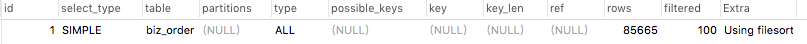• ## 索引命中规则详解

千次阅读 2019-06-30 15:10:00
索引命中规则详解： t这张表 a,b,c 三个字段组成组合索引 索引命中规则详解： t这张表 a,b,c 三个字段组成组合索引 select * from t where a=? and b=? and c=? 全命中 select * from t where c=? and b=? and...
索引命中规则详解：

t这张表 a,b,c 三个字段组成组合索引

索引命中规则详解：

t这张表 a,b,c 三个字段组成组合索引

select * from t where a=? and b=? and c=?  全命中

select * from t where c=? and b=? and a=?  全命中 解析MySQL的查询优化器会自动调整where子句的条件顺序以使用适合的索引

select * from t where a=?  命中a  解析:最左前缀匹配

select * from t where a=? and b=?  命中a和b  解析:最左前缀匹配

select * from t where a=? or b=?  一个没命中 解析or无法命中

select * from t where a=? and c=?  命中a 解析:最左前缀匹配，中间没有则无法使用索引

select * from t where a=? and b in ( x, y, z) and c=?  全部命中 in精确匹配可以使用索引

select * from t where b=?  一个没命中  解析:最左前缀匹配原则

select * from t where b=? and c=?  一个没命中  解析:最左前缀匹配原则

select * from t where a=? and b like 'xxx%'   命中a

select * from t where a=? and b like '%xxx'  命中a和b

select * from t where a<? and b=?   命中a 解析这个是范围查找

select * from t where a between ? and ?  and b=?  命中a和b 解析BETWEEN相当于in操作是精确匹配

select * from t where a between ? and ?  and b=? and c  and between ? and ?    全部命解析中同上

select * from where a-1=?   函数和表达式无法命中索引


展开全文mysql索引 索引 最左前缀匹配
• mysql 索引命中规则多列索引 遵循：最左匹配原则不会命中索引的情况 当一条sql语句提交给mysql数据库进行查询的时候需要经历以下几步 1、先在where解析这一步把当前的查询语句中的查询条件分解成每一个独立的条件...
mysql 索引命中规则 不命中的情况多列索引 遵循：最左匹配原则不会命中索引的情况案例分析
当一条sql语句提交给mysql数据库进行查询的时候需要经历以下几步
1、先在where解析这一步把当前的查询语句中的查询条件分解成每一个独立的条件单元
2、mysql会自动将sql拆分重组
3、然后where条件会在B-tree index这部分进行索引匹配，如果命中索引，就会定位到指定的table records位置。如果没有命中，则只能采用全部扫描的方式
4、根据当前查询字段返回对应的数据值

多列索引 遵循：最左匹配原则
因此在当进行以下查询时仍会命中mysql索引
select * from student where userName=‘小明’;
select * from student where userName=‘小明’ and age=19;
select * from student where userName=‘小明’ and age=19 and phone=‘1887821’;

不会命中索引的情况

如果where后面有or，这样不会命中索引如：
select * from student where userName=‘小明’ or age=19;
如果想要or后面的也走索引的话，应该是创建两个单列索引

like是以%开头的不会命中索引如：
select * from student where userName like %明

如果列类型是字符串，那一定要在条件中将数据使用引号引用起来,否则不使用索引如：
select * from student where userName=‘小明’ and age=19 and  phone=1887821 ;
phone 定义的数据格式是字符串，但是sql中使用的整型

没有查询条件，或者查询条件没有建立索引

查询条件中，在索引列上使用函数（+/-*/）
select * from student where userName=‘小明’ and age-1=19 错误
select * from student where userName=‘小明’ and age=20   正确

采用 not in, not exist，!=, <> , is null ,   is not null   不会命中索引

案例分析
表有3个普通索引

KEY idx_channel (channel),
KEY idx_store_id (store_id),
KEY idx_clue_id (clue_id)

explain select * from biz_order where store_id = 20036explain select * from biz_order where store_id = 20036 order by channelexplain select * from biz_order order by channelexplain select * from biz_order where store_id = 20036 group by clue_id order by channel可看到通过group by命中了idx_clue_id索引, order by 未命中索引


展开全文mysql 索引
• mysql索引命中规则 当我们有一条SQL： select * from student where name=‘张三’ and age=15 and phone=‘13873062211’; 创建一个简单的单列索引： alter table student add index name_index (name); 将name列建...
mysql索引命中规则
当我们有一条SQL：
select * from student where name=‘张三’ and age=15 and phone=‘13873062211’;
创建一个简单的单列索引：
alter table student add index name_index (name);
将name列建索引，这样就把范围限制在name='张三’的结果集上，之后扫描结果集，产生满足age=15的结果集，再扫描该结果集，找到 phone='13873062211’的结果集，即最终结果。


展开全文mysql 数据库
• 索引命中规则详解： t这张表 a,b,c 三个字段组成组合索引 select * from t where a=? and b=? and c=? 全命中 select * from t where c=? and b=? and a=? 全命中 解析MySQL的查询优化器会自动调整where子句的条件...
索引命中规则详解：
t这张表 a,b,c 三个字段组成组合索引
select * from t where a=? and b=? and c=?  全命中
select * from t where c=? and b=? and a=?  全命中 解析MySQL的查询优化器会自动调整where子句的条件顺序以使用适合的索引
select * from t where a=?  命中a  解析:最左前缀匹配
select * from t where a=? and b=?  命中a和b  解析:最左前缀匹配
select * from t where a=? or b=?  一个没命中 解析or无法命中
select * from t where a=? and c=?  命中a 解析:最左前缀匹配，中间没有则无法使用索引
select * from t where a=? and b in ( x, y, z) and c=?  全部命中 in精确匹配可以使用索引
select * from t where b=?  一个没命中  解析:最左前缀匹配原则
select * from t where b=? and c=?  一个没命中  解析:最左前缀匹配原则
select * from t where a=? and b like ‘xxx%’   命中a
select * from t where a=? and b like ‘%xxx’  命中a和b
select * from t where a<? and b=?   命中a 解析这个是范围查找
select * from t where a between ? and ?  and b=?  命中a和b 解析BETWEEN相当于in操作是精确匹配
select * from t where a between ? and ?  and b=? and c  and between ? and ?    全部命解析中同上
select * from where a-1=?   函数和表达式无法命中索引


展开全文mysql
• ## Oracle 索引命中详解

千次阅读 2019-06-21 16:27:51
整理一下Oracle数据查询索引命中情况，仅供参考，有问题欢迎指出修正： 创建测试表： CREATE TABLE TEST_HZ ( A VARCHAR(20), B VARCHAR(20), C VARCHAR(20) )； 插入数据： 本人比较懒，然后就直接建序列插...Oracle
• 如何查看mongodb的索引命中率 一、背景 现在mongodb使用率很高，经常会遇到查询慢时，就会创建索引，而有时候索引命中率又不高，下面来介绍下测试环境下如何查看索引命中率 二、方案 1、首先查看查询collection的...mongodb
• 索引命中情况、索引命中类型、命中率等相关的信息,通过这种方式 可以对sql语句进行调优,对关键查询的条件创建索引以及注意一下sql语句的用法 尽量不使用like,尽量避免在某一列上进行运算,不使用 in not in 使用exist...mysql sql 数据库 java
• 2、等值的条件去命中索引最左边的一个字段，然后依次从左往右命中，范围的放在最后。 分析讲解 1、mysql的索引分为聚簇索引和非聚簇索引，mysql的表是聚集索引组织表。 聚集规则是：有主键则定义主键索引为聚集...mysql
• t这张表 a,b,c 三个字段组成组合索引 select * from t where a=?... 全命中 解析MySQL的查询优化器会自动调整where子句的条件顺序以使用适合的索引 select * from t where a=? 命中a 解析:最左前缀匹配...
• 1.like前缀命中，如 xx% ,,而%xx%,%xx 无法命中索引 2.or 应该无法命中索引 3.在where语句中 使用!= 或< >这种操作符，无法命中索引 4.where语句中，做null值判断，无法命中 5.组合索引的话，如(a,b,c)上建立...
• 有多少是命中索引的?命中哪个索引?索引中有哪个是无效索引?这些无效索引是否会影响系统的性能?带着这些问题我们一起来学习一下.　MySql中是通过 Explain 命令来分析低效SQL的执行计划。命令的使用很简单.示例 ...
• ## MYSQL索引命中率

千次阅读 2019-04-07 14:45:29
一、MySQL 索引类型 1、从物理存储角度上，索引可以分为聚集索引和非聚集索引。 ①.聚集索引（Clustered Index） 聚集索引决定数据在磁盘上的物理排序，一个表只能有一个聚集索引。 ②. 非聚集索引（Non-...索引 mysql 优化 MySQL优化
• 和a、c情况到底会不会命中索引？ 网上查阅相关博客发现很多答案不一样，于是我干脆亲手操作实验一下 一:创建表 为了更直接贴合面试题，字段直接用AA,BB,CC表示 create table IF NOT EXISTS TEST_COMPOSITE_INDEX ( ...mysql java
• ## Mysql索引命中问题

千次阅读 2018-11-09 15:33:24
索引概念和作用   索引是一种使记录有序化的技术，它可以指定按某列/某几列预先排序，从而大大提高查询速度（类似于汉语词典中按照拼音或者笔画查找）。   索引的主要作用是加快数据查找速度，提高数据库的...mysql
• 1.两种不会命中索引，导致全表扫描情况： 第一类：“列类型”与“where值类型”不符，不能命中索引，会导致全表扫描(full table scan)。 数据准备： create table t1 ( cellvarchar(3) primary key )engine=...
• ## mysql索引命中分析

千次阅读 2017-11-30 14:18:39
本文将告知mysql的索引类型，sql的依据，什么时候会使用索引，什么情况不会使用索引mysql...